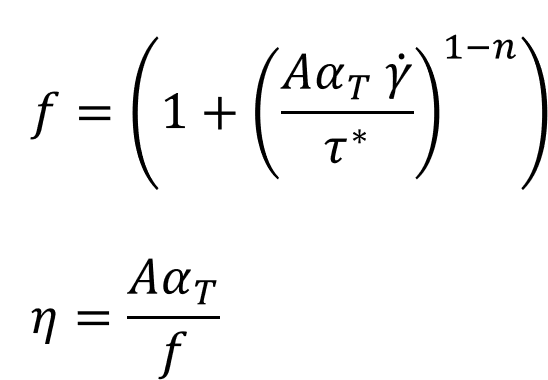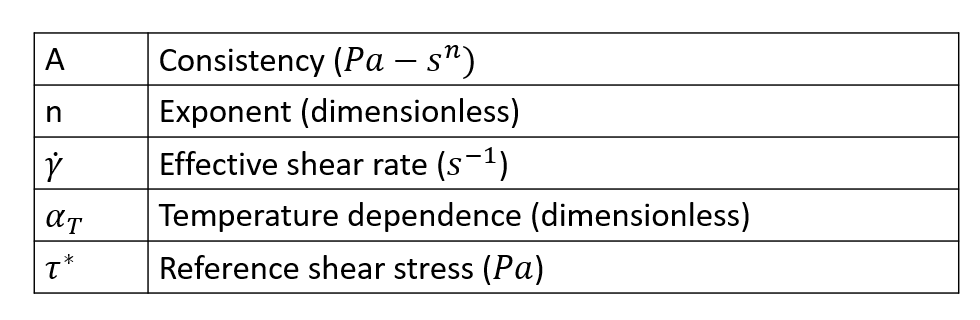# Modified Cross Model

Modified Cross model is expressed by the following equation. This model has three parameters: consistency A, exponent n, and reference shear stress t*. Consistency is modified using the temperature dependent function a_T to introduce the effect of temperature on the computed viscosity. There is a very subtle difference between Cross and Modified Cross model, this difference enables (by appropriate choice of parameters) one to easily set up Carreau-Yasuda model to mimic the modified Cross model.This model describes the viscosity using a power-law relationship. The factor (A alpha_T/tau*) is like a time constant in the equation. Depending on the value of the exponent used, the nature of the model will change.

n < 1 Shear thinning or Pseudoplastic

n > 1 Shear thickening or dilalatant

n = 1 Newtonian

For most polymers, Exponent n is less than 1. When the exponent is less than 1, viscosity of the polymer decreases with increase in the shear rate. This behavior is called shear thinning. On the other hand, when the exponent is greater than 1, viscosity increases with shear rate and this behavior is called shear thickening. Refer to the section Power Law Model for a detailed discussion of this concept.

## Explanation of Parameters

Parameter Description Units Data Type Condition Typical Value
ConstitutiveModel Describes the model used None String Required "ModifiedCross"
Density Density of the polymer kg/m^3 Constant Required 995.0
SpecificHeat Specific heat at constant pressure J/kg/K Constant/F(T) Required 2000.0
Conductivity Thermal conductivity W/m?k Constant/F(T) Required 0.167
CoeffOfThermalExpansion Indicates the change in volume with change in temperature 1/K Constant Required 1.0e-05
VolumetricHeatSource Heat generated/removed in the volume by methods like elctrical heating W/m63 Constant Required 0.0
Consistency One of the parameters of the modified Cross model Pa Constant Required 0.01
Exponent Power law index, defines the dependency of viscosity on shear rate. None Constant Required 0.66
ReferenceShearStress One of the parameters of the modified cross model. Pa Constant Required 75000
ZeroShearRateLimit Defines the trunctation limit for shear rate. See Power law model. 1/s Constant Required 0.01
TemperatureDependence See temperature Dependence None string Required "WLF"
ReferenceTemeprature Temperature at which data is calculated for the initialization step. K Constant Required only if TD is not "None" 533
FreezeTemperature This is the no flow temperature. Below this temperature, material ceases to flow. K Constant Required only if TD is not "None" 350
ActivationEnergy A parameter required by Arrhenius model. J/mol Constant Required only if Td is Exp(Q/RT) 16628
UniversalGasConstant A parameter from state equation PV = nRT, R is universal gas constant J/mol/K Constant Required onlyh if TD is Exp (Q/RT) 8.314
TemperatureSensititvy A derived parameter which has the same physical meaning as Q/R. K Constant Required only if TD is Exp(Tb/T) 2000 K
WLFConstant1 Constant C1 of WLF model none Constant Required only if TD is WLF 17.44
WLFConstant2 Constant C2 of WLF model. This is like DeltaT, hence the value is same in K and Celsius. K Constant Required only if TD is WLF 51.6
GlassTransitionTemperature Temperature below with polymer molecules ceases to move (frozen). There are few definitions of this term. K Constant Required only if TD is WLF 320
Beta Parameter in the relationship Exp(-Beta(DeltaT)) None Constant Required only if TD is exp(-Beta(Delta T)) 0.005